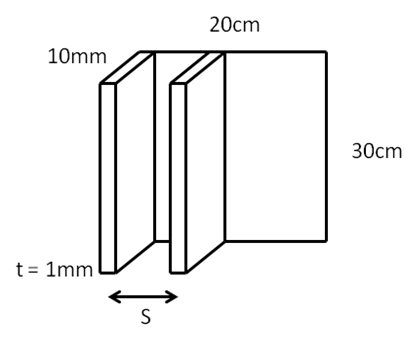# Heat Sink Design

## Heat Sink Design

In last month’s Blog we discussed alternative techniques for increasing the amount of heat dissipation in electronic circuits and components. One of the most commonly used approaches to increase heat dissipation is the use of heat sinks. Heat sink design seeks to maximize the surface area in contact with the surrounding cooling medium, generally air in these applications, and is attached using thermal interface materials that have high thermal conductivity and fill the air gap between the component and the heat sink. Air velocity, direction of flow, choice of material, protrusion design and surface treatment are all factors that affect the performance of a heat sink. Heat sink design can take many forms:

1. Plate-fins: A series of plates or sheets run along the entire length of the attachment area
2. Pin-fins: Pins are arranged in a regular array and extend
3. Flared plate fins: A series of non-parallel plates that run along the entire length of the attachment area

In this Blog we address heat sink design and provide guidelines for developing an optimum design for the case of natural convection.

### How to design a Heat Sink

A heat sink transfers thermal energy from a higher temperature device to a surrounding fluid medium which is generally air but could also be water, oil or a refrigerant depending on the application, for the purposes of the applications of interest here we will consider the fluid to be air. Thermal dissipation through the heat sink occurs by a conjugate heat transfer mechanism in which transfer of thermal energy occurs by a combination of conduction through the heat sink and into the fluid, convection of the surrounding fluid and radiation into the environment. In most situations, heat transfer across the interface between the solid surface and the coolant air is the least efficient within the system, and the solid-air interface represents the greatest barrier for heat dissipation, a heat sink lowers this barrier mainly by increasing the surface area that is in direct contact with the coolant.

Natural convection arises because the hot air has a lower density and thus rises under the buoyancy forces created. This flow can be represented by the dimensionless Grashof number, Gr, that represents the ratio of the buoyancy force to the viscous force acting on the fluid and is given by:

Gr        ~ Buoyancy force / Viscous forces

= g.β.(Ts-T).δ3 / ν2                 (1)

Where g is the gravitational acceleration (ms-2), β is the coefficient of volume expansion (1/K), Ts is the surface temperature (K), T is the ambient temperature (K), δ is the characteristic length of the geometry (m), and ν is the kinematic viscosity of the fluid (m2s-1). For vertical plates values of Gr > ~ 109 flow is turbulent.

Heat transfer into the fluid can be characterized by the Prandtl number, Pr, momentum diffusivity to thermal diffusivity:

Pr         = viscous diffusion rate / thermal diffusion rate

= (cp.µ) / k                               (2)

Where cp is the specific heat capacity (J/kg.K), µ is the dynamic viscosity (Pa.s) and k is thermal conductivity (W/m.K). The Prandtl number controls the relative thickness of the momentum and thermal boundary layers and is often tabulated with other fluid properties, for air and many other gases it is considered that Pr ranges between 0.7 and 0.8. When heat conduction is effective compared to convection: thermal diffusivity is dominant. When convection is effective in transferring energy from an area, compared to pure conduction: momentum diffusivity is dominant.

The optimum heat sink design is a balance of maximizing surface area while at the same time setting the spacing of the fines to allow maximum air flow and minimizing friction. Thus heat sinks with closely spaced fins are not suitable for natural convection. The optimum fin spacing for a vertical heat sink is given by:

Sopt = 2.714 (L/Ra1/4)                            (3)

Where L is the characteristic length and Ra is the Reynolds number.

The heat transfer coefficient for the optimum spacing is given by:

H = 1.31 (k/Sopt)                                   (4)

Consider the following example: A vertical surface at 80°C is to be cooled by a heat sink in air at an ambient temperature of 20°C. The plate has a width of 20 cm and a height of 30cm, the fins of the heat exchanger are 1mm thick, 30cm long and have a height of 10mm.The average temperature of the air on the surface of the fin is 50°C: at this temperature the thermal conductivity, k, is 0.0279 W/m.K, the kinematic viscosity, ν, is 1.82 x 10-5 m2/s, Pr is 0.709 and assuming ideal gas behavior the coefficient of volume expansion, β, is 1/(Ts+T)/2) = 3.1 x 10-3. The characteristic length , L, is the length of the fin thus the Reynold’s number, Ra, is given by:

Ra        = Gr.Pr

The optimum spacing Sopt is then determined by equation 3 to be 8.03mm. For a fin thickness of 1mm the number of fins on the heat exchanger is ~22. The heat transfer coefficient can be calculated using equation 4 to be 4.54 W/m.K.